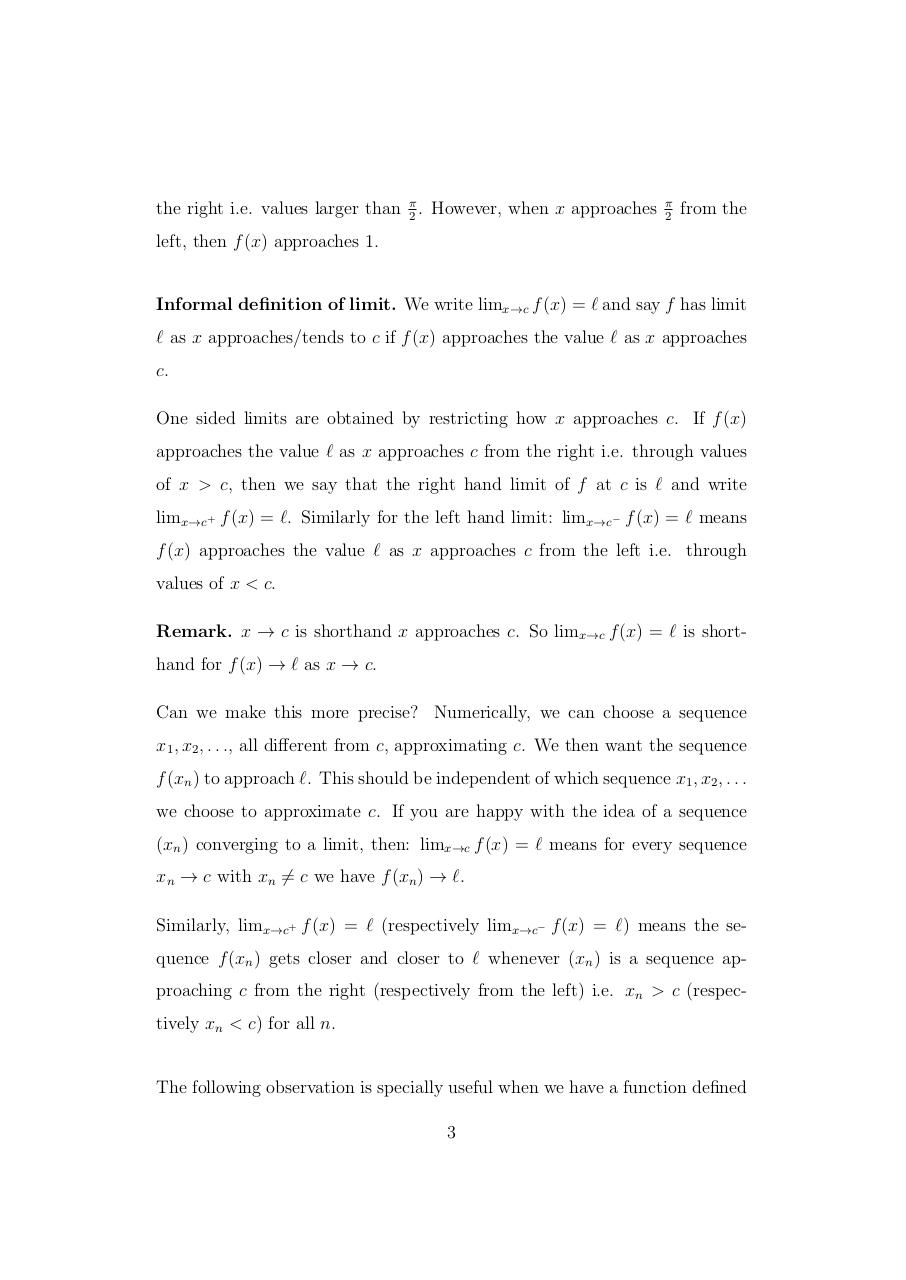# Limits.pdfPage 1 2 3 4 5 6 7 8 9 10 11 12

#### Text preview

the right i.e. values larger than π2 . However, when x approaches

π
2

from the

left, then f (x) approaches 1.

Informal definition of limit. We write limx→c f (x) = ` and say f has limit
` as x approaches/tends to c if f (x) approaches the value ` as x approaches
c.
One sided limits are obtained by restricting how x approaches c. If f (x)
approaches the value ` as x approaches c from the right i.e. through values
of x &gt; c, then we say that the right hand limit of f at c is ` and write
limx→c+ f (x) = `. Similarly for the left hand limit: limx→c− f (x) = ` means
f (x) approaches the value ` as x approaches c from the left i.e. through
values of x &lt; c.
Remark. x → c is shorthand x approaches c. So limx→c f (x) = ` is shorthand for f (x) → ` as x → c.
Can we make this more precise? Numerically, we can choose a sequence
x1 , x2 , . . ., all different from c, approximating c. We then want the sequence
f (xn ) to approach `. This should be independent of which sequence x1 , x2 , . . .
we choose to approximate c. If you are happy with the idea of a sequence
(xn ) converging to a limit, then: limx→c f (x) = ` means for every sequence
xn → c with xn 6= c we have f (xn ) → `.
Similarly, limx→c+ f (x) = ` (respectively limx→c− f (x) = `) means the sequence f (xn ) gets closer and closer to ` whenever (xn ) is a sequence approaching c from the right (respectively from the left) i.e. xn &gt; c (respectively xn &lt; c) for all n.
The following observation is specially useful when we have a function defined
3